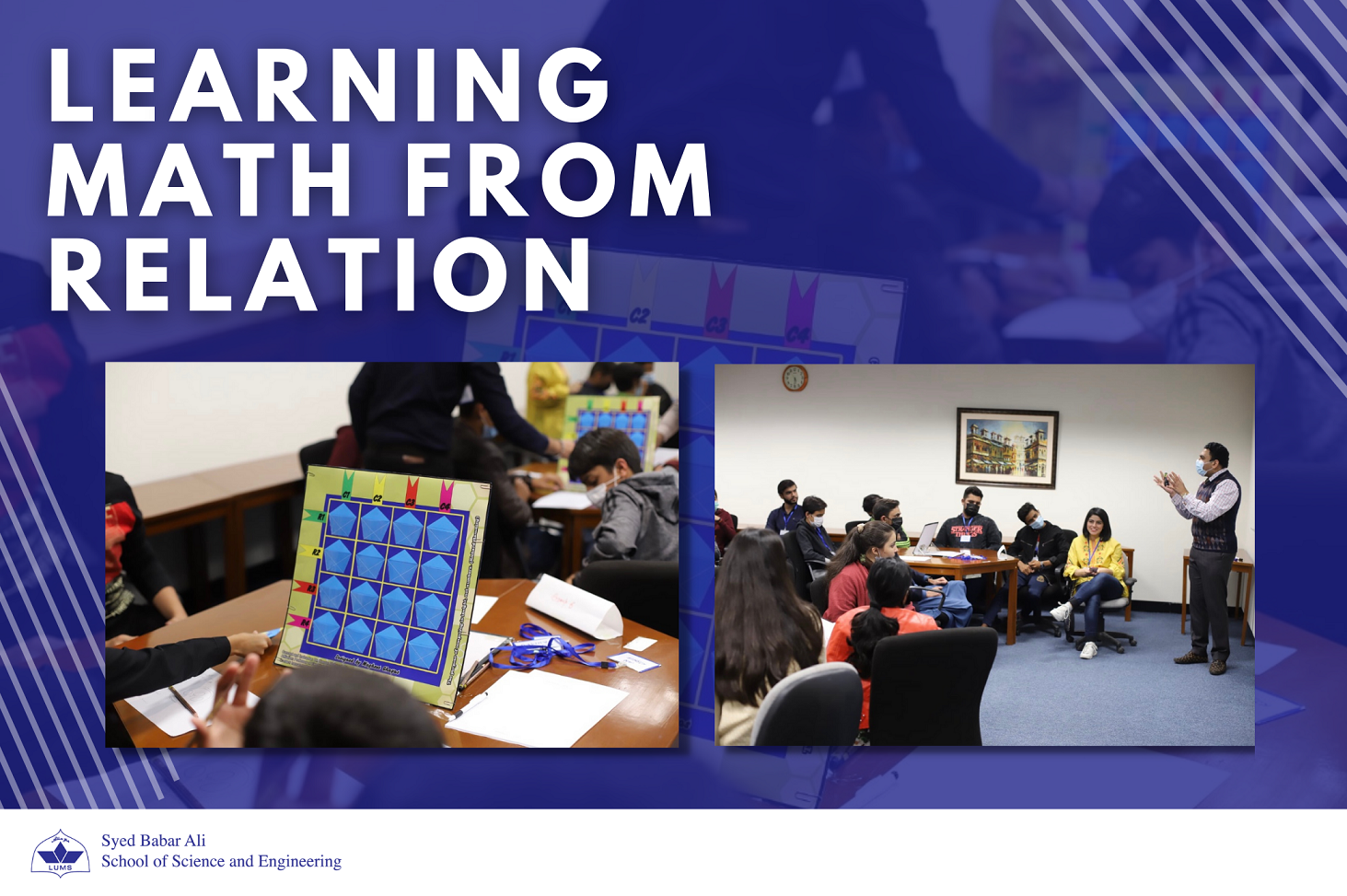Post Date
Dec 20 2021

## Learning Math from Relation

Authors
Hira Shariq

I remember when I was in 7th grade, just starting out algebra. It was chaos. The x’s and y’s made no sense. My classmates and I just couldn’t wrap our heads around why was this even something to study. And it was an astonishing realization that math will get tougher along the years.

Recently, Dr. Hassan Mohy Ud Din, Assistant Professor at the Electrical Engineering Department at SBASSE LUMS, hosted his Math Circle, “Reconstruction of the Unknown”. The seminar made me wonder if assigning physical context to theoretical mathematics would make it easier for students to find x. Math Circles are hosted by the Math Department at LUMS for middle school and high school students, belonging to different kinds of schools and school systems. Dr. Mohy Ud Din’s was the third in the series. He decided to teach them matrices but in a way that all could understand, regardless of their background. What was the result? Simultaneous equations made easy!

He explained this concept by ensuring that students were able to visualize a matrix and its operations using their experience. At first, the professor explained how really, while computing only two math operations are required. He testified that subtraction is just addition of a negative number, and that division is just multiplication of an inverse of the number. Presenting this idea, Dr. Mohy Ud Din was able to make his audience realize how

Next students were shown graphics of a person walking, forward and backward, what the professor called was sweeping the x-axis whilst performing horizontal motion. Then, similarly, he showed students how a person climbing, up and down, was sweeping the y-axis whilst doing vertical motion. He showed that these two motions each represented one-dimension motion by presenting them an image of a Cartesian graph. Students realized that using both the x-axis and y-axis together, a two-dimension can be described. To help them visualize, Dr. Hassan narrated them Anand’s Immortal, the famous chess game from 2013. He showed them how 2D representation in chess is like that in a typical matrix. Rows and columns are used together to locate the numbers in the matrix, just like how chess players memorize names of locations on the chess board.

The star of the program was Dr. Mohy Ud Din’s board game. The audience was divided into groups and each group was divided into two subgroups. Amongst the group, one of the subgroups was given a 4-by-4 matrix type board, and a stack of cards that had numbers 0-13. That subgroup randomly picked 4 cards and made a 2-by-2 matrix. The other subgroup guessed what those 4 numbers are. But they didn’t have to the guess casually! They were told the sums of each column and sums of each row. Then they must simultaneously solve the equations to get their answers. They did the same task with a 3-by-3 matrix, but it could not be solved! Students realized that to solve a system, the number of equations should be equal to the number of unknows. In a 3-by-3 system, the number of equations (3 for the rows and 3 for the columns) were lesser than the number of unknowns (nine). Amazingly, students suggested that they could use diagonal sums. Dr. Hassan realized that diagonals were a primary grade math concept that the students were able to connect to themselves. This made it easy for the professor to explain an upper triangular matrix, which led to students themselves wondering about a lower triangular matrix. Then, he explained sparse and dense matrices that he related to sparsely and densely populated areas.

Dr. Mohy Ud Din believes that such seminars can complement traditional education to keep the motivation and sharpen a student’s observation. They will be visualizing and using their memory by making relations to their experience to learn math!